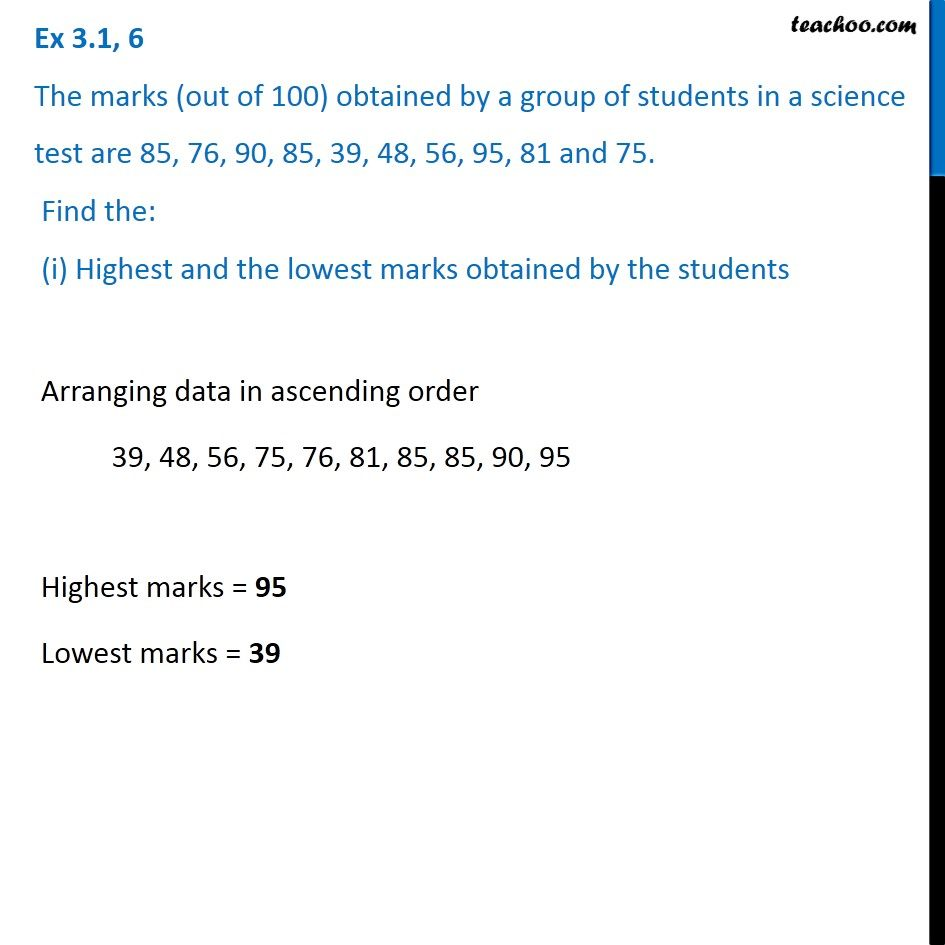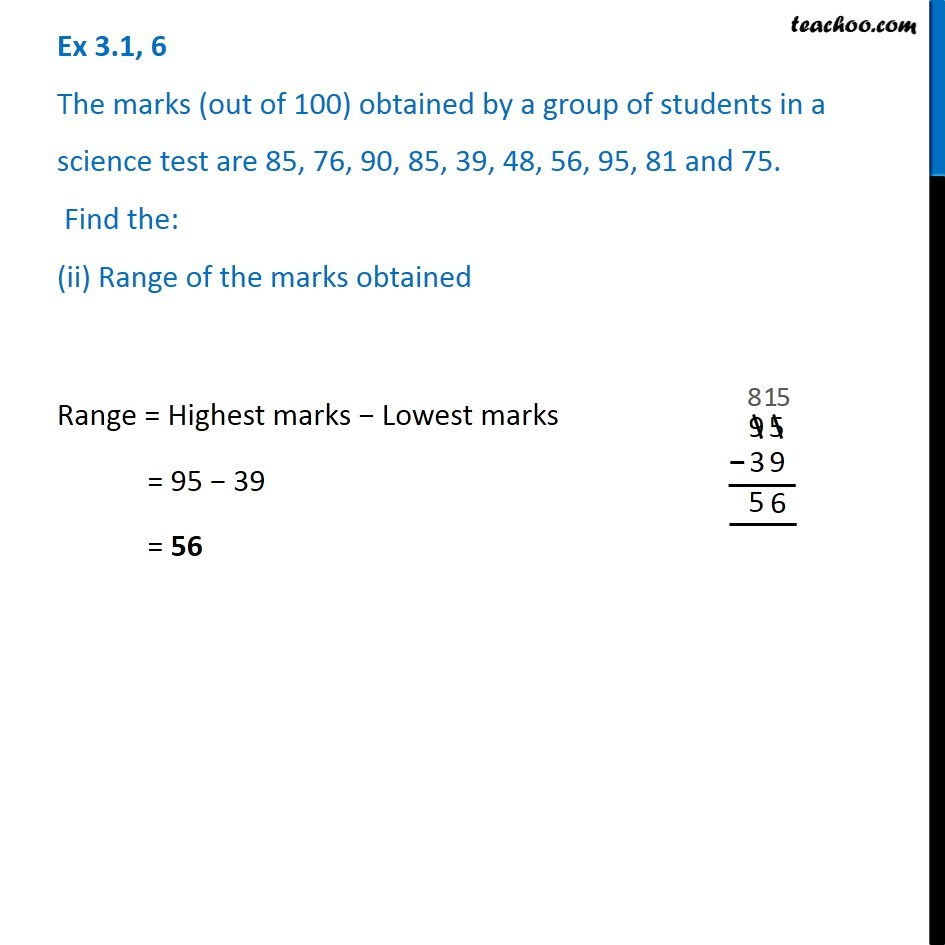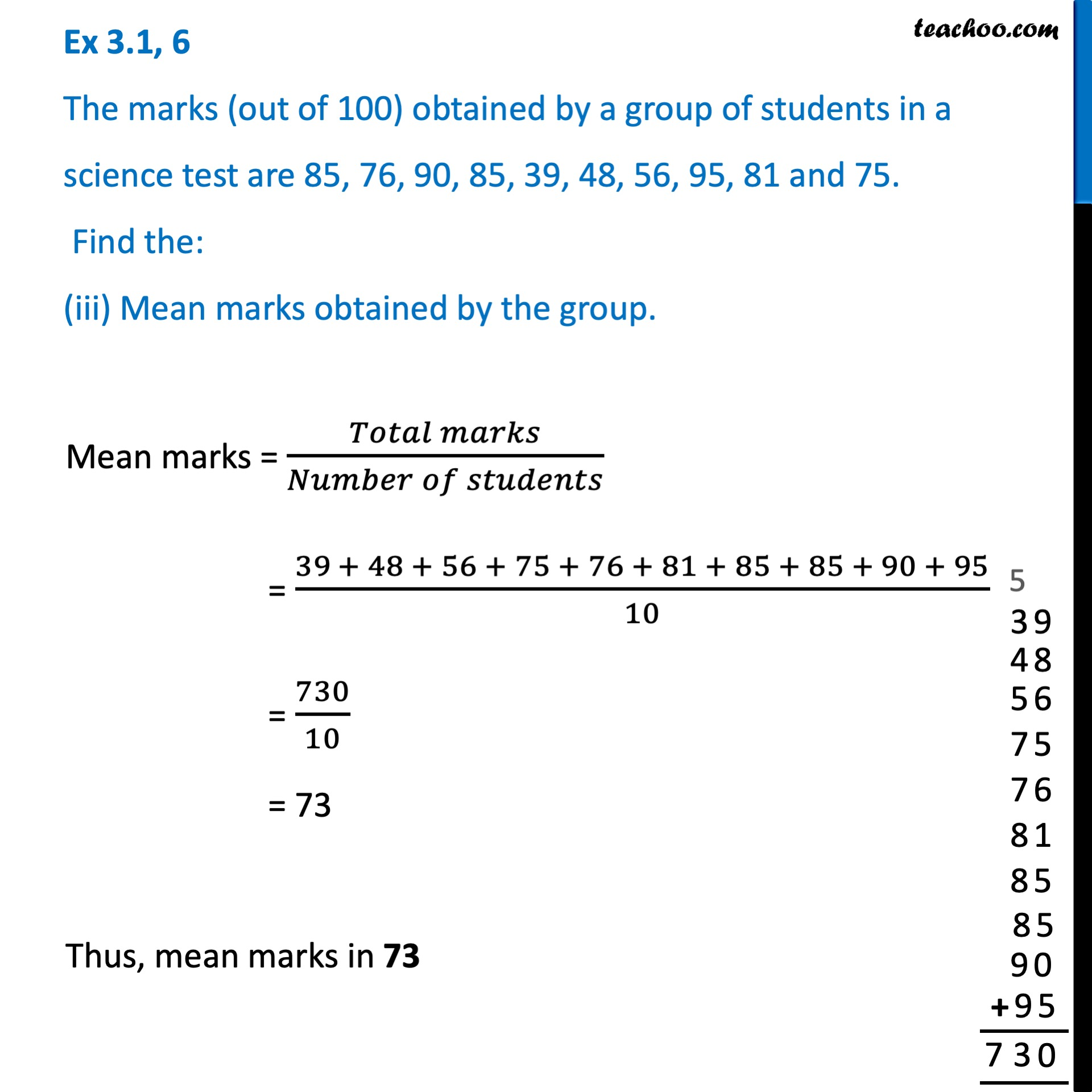Ex 3.1

Chapter 3 Class 7 Data Handling
Serial order wiseLearn in your speed, with individual attention - Teachoo Maths 1-on-1 Class

### Transcript

Ex 3.1, 6 The marks (out of 100) obtained by a group of students in a science test are 85, 76, 90, 85, 39, 48, 56, 95, 81 and 75. Find the: (i) Highest and the lowest marks obtained by the students Arranging data in ascending order 39, 48, 56, 75, 76, 81, 85, 85, 90, 95 Highest marks = 95 Lowest marks = 39 Ex 3.1, 6 The marks (out of 100) obtained by a group of students in a science test are 85, 76, 90, 85, 39, 48, 56, 95, 81 and 75. Find the: (ii) Range of the marks obtained Range = Highest marks − Lowest marks = 95 − 39 = 56 Ex 3.1, 6 The marks (out of 100) obtained by a group of students in a science test are 85, 76, 90, 85, 39, 48, 56, 95, 81 and 75. Find the: (iii) Mean marks obtained by the group. Max marks = (𝑇𝑜𝑡𝑎𝑙 𝑚𝑎𝑟𝑘𝑠)/(𝑁𝑢𝑚𝑏𝑒𝑟 𝑜𝑓 𝑠𝑡𝑢𝑑𝑒𝑛𝑡𝑠) = (39 + 48 + 56 + 75 + 76 + 81 + 85 + 85 + 90 + 95)/10 = 730/10 = 73 Thus, mean marks in 73